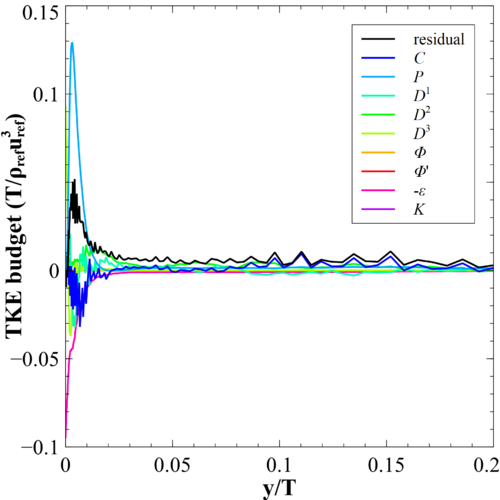# Quantification of resolution

This section provides details of the solution accuracy obtained by tackling the wing-body junction DNS with MIGALE. After providing details of the mesh resolution in comparison with spatial turbulent scales, a discussion on the closure of the Reynolds stress budgets is given.

## Mesh resolution

The mesh resolution is quantified by comparing the characteristic mesh length (${\Delta }$) with the characteristic lengths of the turbulence, i.e., the Taylor microscale (${\eta _{T}}$) and the Kolmogorov length scale (${\eta _{K}}$). Here, the characteristic mesh length takes into account the degree of the DG polynomial approximation. In particular, it is defined as the cubic root of the ratio between the mesh element volume $\left(V\right)$and the number of DoFs $\left(N_{DoF}\right)$within the mesh element per equation

$\Delta ={\sqrt[{3}]{\dfrac {V}{N_{DoF}}}}$To assess the mesh resolution, five planes have been extracted within the highly resolved region of the turbulent flow, see Fig. 7. Planes A and B are parallel to the horizontal solid wall ($y/T=0$) and are placed at $y/T=0.0025$and $y/T=0.1$, respectively. Planes C and D are perpendicular to the streamwise direction and are placed at $x/T=0.75$(location of maximum wing thickness) and $x/T=4.5$(behind the wing trailing edge), respectively, where $x/T=0$is the streamwise coordinate of the wing leading edge. Plane E is the test case geometric symmetry plane ($z/T=0$).

The comparison with respect to the Taylor microscale is shown in Fig. 8. For all the planes extracted, the ratio ${\Delta }/{\eta _{T}}$is lower than 0.6. Accordingly, the current space resolution is sufficient to capture the turbulence scales in the intertial range.

In Fig. 9 is reported the comparison with respect to the Kolmogorov length scale. It is commonly accepted that DNS requirements are satisfied when ${\Delta }/{\eta _{K}}\leq 5$. In all planes considered there is clearly visible a region around the wing in which the ratio ${\Delta }/{\eta _{K}}$is greater than 8, but no larger than 10. This region is characterized by the presence of the horse-shoe vortex. Besides, for planes D and E an additional region of high ${\Delta }/{\eta _{K}}$ratio can be seen downstream of the wing trailing edge, close to the symmetry plane. This is the region where the turbulent boundary layer that develops along the wing solid wall moves downstream and generates a wake. As outcome of this analysis, it can be stated that the DNS requirements are not fulfilled for the current simulation. For this reason the present study was referred to as under-resolved DNS (uDNS). For future highly resolved simulations, further mesh refinement is strongly advised in these regions. Notice that the accurate simulation of the wake behind the wing away from the floor was not within the scope of the current computational campaign. The low mesh resolution in this region was indeed expected as the computational grid had to be coarsened along the vertical (normal to the floor) direction due to computational cost constraints.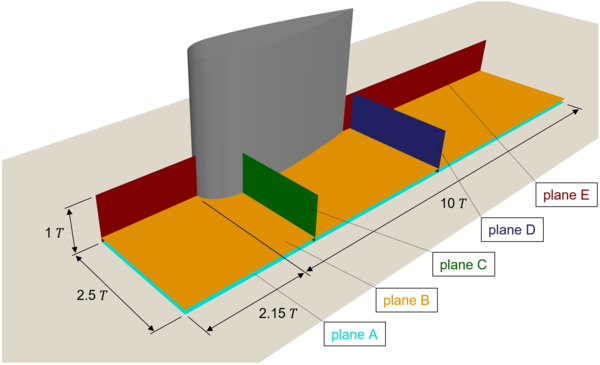Figure 7: Wing-body junction. Extracted planes for mesh resolution analisys.

The average near-wall resolution in streamwise (${\Delta x^{+}}$), spanwise (${\Delta z^{+}}$) and wall-normal (${\Delta y^{+}}$) directions at different locations on the horizontal solid wall is reported in Tab. 3.

 ${x/T}$${z/T}$${\Delta x^{+}}$${\Delta z^{+}}$${\Delta y_{1}^{+}}$${-2.15}$${0}$${23.3}$${21.6}$${0.46}$${2.125}$${0.75}$${32.4}$${41.9}$${0.53}$${4.5}$${0.5}$${22.2}$${15.3}$${0.51}$${8.5}$${1}$${24.6}$${19.1}$${0.43}$Table 3: Near-wall space resolution at different locations. $(x/T,z/T)=(0,0)$is the wing leading edge - horizontal solid wall intersection point

## Solution verification

One way to verify that the DNS are properly resolved is to examine the terms in the Reynolds-stress and turbulent kinetic energy (TKE) equation (the budgets).

As a first step, an assessment of code MIGALE in closing the budgets is performed. Fig. 10 reports the budgets of streamwise normal Reynolds-stress and TKE in a channel flow at ${Re_{\tau }=180}$using a DG polynomial approximation of degree 5 on a mesh of $91\times 43\times 48$hexahedral elements (10.5 million DoF/eqn.). Domain dimensions are those of the DNS of Moser et al. (1999) and their results are included as reference. As the maximum value of the residual is $0.22\%$and $0.64\%$of the production peak for the Reynolds-stress xx and the TKE budgets, respectively, the results show that the MIGALE code can close well the budgets when sufficient spatial and time resolution is used.

 Figure 10: Channel flow at $Re_{\tau }=180$Reynolds-stress xx and TKE budgets: dissipation $\varepsilon$, production $P$, turbulent diffusion $D^{1}$, pressure diffusion $D^{2}$, viscous diffusion $D^{3}$and pressure strain $\Phi$. Solid lines from the DG P5 computation and symbols from Moser et al. (1999).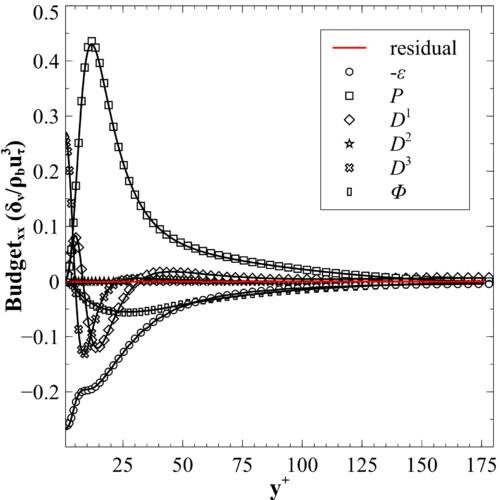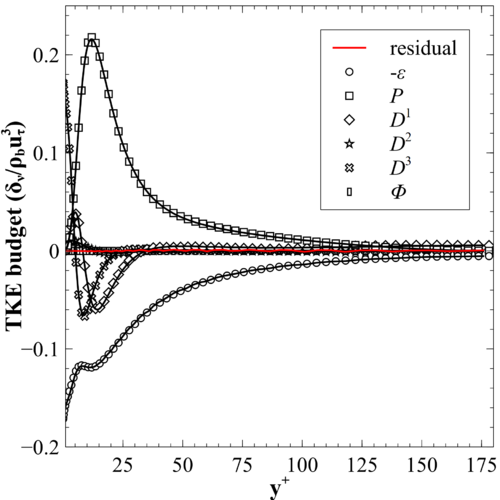Considering now the budgets for the wing-body junction simulation, the conclusion obtained comparing the characteristic mesh length and the Kolmogorov length scale (see Fig. 9) still holds. Indeed, on Fig. 11 and Fig. 12 are reported the TKE budgets for two different locations, i.e., $(x/T,z/T)=(-2.15,0)$(the chekpoint streamwise location) and $(x/T,z/T)=(2.125,0.75)$(on the side of the wing, in the region of the horse-shoe vortex), respectively. In both locations the budget closure has not been achieved. The oscillating behaviour of the profiles of the different terms can be ascribed to a lack of spatial resolution. This result suggests that for future campaigns it is recommended to increase further the grid density.

Figure 11: Wing-body junction. TKE budgets at location $x/T=-2.15$, $z/T=0$: convection $C$, production $P$, turbulent diffusion $D^{1}$, pressure diffusion $D^{2}$, viscous diffusion $D^{3}$, pressure strain $\Phi$, pressure dilatation $\Phi '$, dissipation $\varepsilon$and density fluctuation $K$.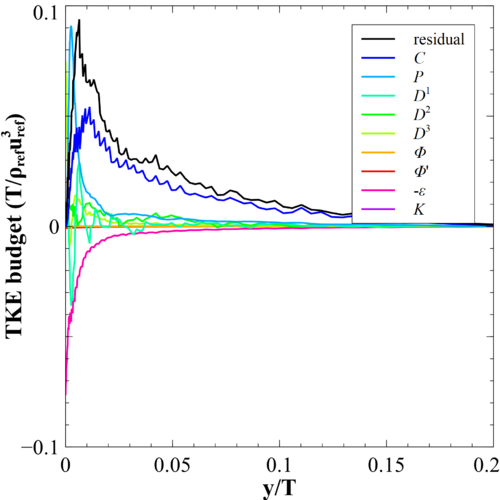Figure 12: Wing-body junction. TKE budgets at location $x/T=2.125$, $z/T=0.75$: convection $C$, production $P$, turbulent diffusion $D^{1}$, pressure diffusion $D^{2}$, viscous diffusion $D^{3}$, pressure strain $\Phi$, pressure dilatation $\Phi '$, dissipation $\varepsilon$and density fluctuation $K$.